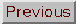### VIRIAL THEOREM

Adapted from P. Coles, 1999, The Routledge Critical Dictionary of the New Cosmology, Routledge Inc., New York. Reprinted with the author's permission. To order this book click here: http://www.routledge-ny.com/books.cfm?isbn=0415923549

An important result from the field of statistical mechanics that deals with the properties of self-gravitating systems in equilibrium. According to the theory of the Jeans instability, small initial fluctuations grow by virtue of the attractive nature of gravity until they become sufficiently dense to collapse. When such a structure collapses it undergoes what is sometimes called violent relaxation: the material that makes up the structure rapidly adjusts itself so that it reaches a kind of pressure balance with the gravitational forces. The velocities of particles inside the structure become randomised, and the structure settles down into an equilibrium configuration whose properties do not undergo any further change. This process is sometimes called virialisation.

The virial theorem, which applies to gravitationally bound objects of this kind, states that the total kinetic energy T contained in the structure is related to the total gravitational potential energy V by the equation

2T + V = 0

This theorem can be applied to gravitationally bound objects such as some kinds of galaxy and clusters of galaxies, and its importance lies in the fact that it can be used to estimate the mass of the object in question.

Because the motions of matter within a virialised structure are random, they are characterised by some dispersion (or variance) around the mean velocity. If the object is a galaxy, we can estimate the variance of stellar motions within it by using spectroscopy to measure the widths of spectral lines affected by the Doppler shift. If the object is a galaxy cluster, we have to measure the redshifts of all the galaxies in the cluster. The mean redshift corresponds to the mean motion of the cluster caused by the expansion of the Universe; the variance around this mean represents the peculiar motions of the galaxies caused by the self-gravity of the material in the cluster. If the variance of the velocities is written as v2, then the total kinetic energy of the object is simply 1/2 Mv2, where M is the total mass.

If the object is spherical and has the physical dimension R, then the total gravitational potential energy will be of the form -GM2 / R, whereis a numerical factor that measures how strongly the object's mass is concentrated towards its centre. Note that V is negative because the object is gravitationally bound. We can therefore make use of the virial theorem to derive an expression for the mass M of the object in terms of quantities which are all measurable: M = Rv2 /G.

This illustration is very simplified, but illustrates the basic point. More detailed analyses do not assume spherical symmetry, and can also take into account forms of energy other than the kinetic and gravitational energy discussed here, such as the energy associated with gas pressure and magnetic fields. In rich clusters of galaxies, for example, the galaxies are moving through a very hot gas which emits X-ray's: the high temperature of the gas reflects the fact that it too is in equilibrium with the gravitational field of the cluster. Viralisation can produce gas temperatures of hundreds of millions of degrees in this way, and this can also be used to measure the mass of clusters (see X-ray astronomy). A virial analysis by Fritz Zwicky of the dynamics of the relatively nearby Coma Cluster provided the first evidence that these objects contain significant amounts of dark matter. The material in these clusters is sufficient to allow a value of the density parameter of around00.2.

A modified version of the virial theorem, called the cosmic virial theorem, applies on scales larger than individual gravitationally bound objects like galaxy clusters: it allows us to relate the statistics of galaxies' peculiar motions to the density parameter. This method also usually produces an estimated value of00.2, indicating that dark matter exists on cosmological scales, but not enough to reach the critical density required for a flat universe.

FURTHER READING:

Tayler, R.J., The Hidden Universe (Wiley-Praxis, Chichester, 1995). Coles, P. and Ellis, G.F.H., Is the Universe Open or Closed? (Cambridge University Press, Cambridge, 1997).